﻿ Analysis of Polynomial Collocation and Uniformly Spaced Quadrature Methods for Second Kind Linear Fredholm Integral Equations – A ComparisonPublications are Open
Access in this journal
Article Versions
Export Article
• Normal Style
• MLA Style
• APA Style
• Chicago Style
Research Article
Open Access Peer-reviewed

### Analysis of Polynomial Collocation and Uniformly Spaced Quadrature Methods for Second Kind Linear Fredholm Integral Equations – A Comparison

Turkish Journal of Analysis and Number Theory. 2019, 7(4), 91-97. DOI: 10.12691/tjant-7-4-1
Received May 12, 2019; Revised June 19, 2019; Accepted July 13, 2019

### Abstract

This work aims to compare the polynomial collocation method against the uniformly spaced quadrature methods (Trapezoidal, Simpson’s and Weddle’s rules) to solve the non-homogeneous linear Fredholm integral equations of second kind with non-singular kernel. Nystrom’s interpolation technique is used to attain closed form approximations of the solutions obtained by quadrature methods. The formulation and implementation of the methods along with application on various test problems are presented for comparison. The results obtained, in general, highlight some cases where polynomial collocation yields ill-conditioned systems after discretization with same basis set, and reflects the suitability of quadrature rules. The main focus has been to extract exact closed form solutions by the used methods which can be used as replacement of analytical solutions.

### 1. Introduction

Many problems in applied sciences and mathematical physics can be formulated in terms of both differential and integral equations, but latter are preferred for approximation processes as differentiation increases the error while integration decreases it. This is the reason that various classical problems modeled in terms of differential equations can be dealt with more efficiently using equivalent integral equations. 1, 2

Integral equations can be solved by different analytical methods (for detailed discussion, refer to the work of authors 1, 2, 3, 4, 5, 6). Due to the complexities and limitations of analytic methods associated with non-linearity and singularities etc, many numerical techniques have been proposed to obtain approximate solutions to different classes of integral equations. There is no specific numerical method to solve all types of integral equations. Due to this, the construction, implementation and comparison of efficient and reliable numerical methods for the solution of integral equations has been a key topic in mathematical research.

In this paper, equation under discussion is the Fredholm integral equation of second kind: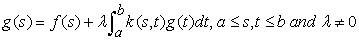(1)

where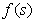and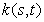are known functions and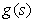is the unknown function to be determined. The functionand the non-zero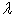(real or complex) are kernel and parameter of (1), respectively. It should be noted that all functions appearing in (1) belong to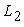space 2.

It is strictly assumed in rest of the paper that in (1),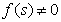(i.e. the non-homogeneous case),is non-singular,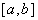is finite and the unknown functioncan be treated with by means of linear operations only. Equation (1) with these specifications can be expressed into equivalent operator form as: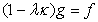(2)

where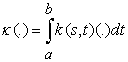(3)

is a bounded linear operator for L2 functions “k” and “g” 2. It should be noted that equation (2) is in scalar form.

Numerous numerical methods have been proposed to solve (1) under specific conditions [7- 9]. One of the important techniques to solve (1) is the polynomial collocation, a projection method, to approximate solution of (1) by a polynomial. This method has been used in 3 and 9 to obtain numerical solution of (1). Moreover, polynomial collocation has also been attempted to solve Volterra integral equations 10, non-linear integral equations 11 and also the initial value problems. 12

Other important methods for the numerical solution of (1) are n-point quadrature schemes. In this work only the uniformly spaced quadrature methods such as, Trapezoidal rule (TR), Simpson’s rule (SR) and Weddel’s rule (WR) are discussed. First two of these have been repeatedly used for the solution of (1) in 3, 13, 14, 15, 16, 17, 18, 19, 20. These methods have also been adopted for the numerical solution of singular and non-linear cases of (1) 21, 22, 23, Volterra integral and integro-differential equations 24, 25, 26 and weakly singular integral equations 27.

In general, TR, SR and WR for the solution of (1) are established by replacing the continuous integral operator “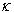” in (2) by a finite n-point uniformly spaced quadrature formula; thus discretizing (2) to a linear system of “ n ” algebraic equations. The resulting linear system is of higher order and, in general, not easily solvable; there can be variety of complexities, as discussed in 28. To approximate solution of the obtained dense system, modified iterative schemes: half sweep and quarter sweep Gauss-Seidel 14, Successive over relaxation 15, Arithmetic mean 16, 17, 18 and Geometric mean 19, 20 methods have been employed in past decade.

Instead of obtaining approximation to the solution of (1) by using methods as in 14, 15, 16, 17, 18, 19, 20, in this work the exact solutions to the dense systems are obtained using MATLAB functions in open form. Nystrom’s technique is then used for interpolation at quadrature points to determine closed form approximations to solution of (1) without having lost already achieved accuracy through quadrature discretization. 29, 30

The paper has been further organized as: Section-2 presents algorithms of methods being used for comparison with necessary assumptions. Section-3 is deals with numerical experiments and implementation of discussed methods to solve some test problems of type (1). The results are discussed and compared in terms of closed form solutions and error plots in Section-4. Finally conclusions are presented in Section-5.

### 2. Material and Methods

We introduce the mathematical analysis of polynomial collocation and Newton-Cotes closed quadrature rules for the numerical solution of (1).

2.1. Polynomial Collocation (Col)

Let “V” be an n-dimensional real linear space of polynomials defined onwith the basis set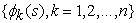. This method approximates the solution to (1) by a polynomial in V which can be expressed as a linear combination of basis polynomials as: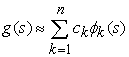(4)

where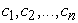are real constants. To make the approach simpler, all basis polynomials are assumed to be exact polynomials in s, that is: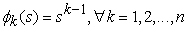(5)

The polynomial collocation approximation of order “n – 1” to solve (1) is: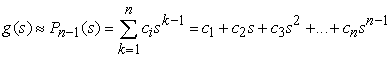(6)

Using (6) in (1) leads to: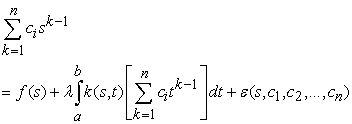(7)

where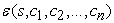is the associated residual depending on s and choice of constants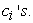For consistent unique determination of coefficients of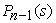, “n” conditions are required. Assuming that the residual “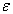” in (7), vanishes at n arbitrarily chosen points: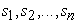, called collocation points, (7) evaluated at the collocation points gives following set of n-equations to determine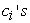: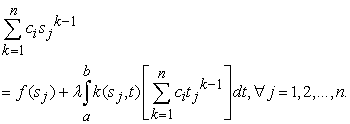(8)

Employing integration in (8), a square system of order n is obtained which can be solved forto finally give (n-1)th order polynomial collocation approximation from (6).

The n-point uniformly-spaced quadrature methods: Trapezoidal rule (TR), Simpson’s rule (SR) and Weddel’s rule (WR) discretize (1) by replacing the bounded linear operator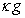in (2) through a weighted sum as: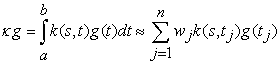(9)

where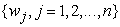is set of weight for the quadrature method being used. Using (9) in (1) leads to: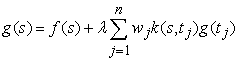(10)

Consider n uniformly-spaced points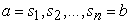, called quadrature points, defined as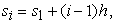where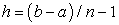and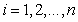, which partition [a, b] into n - 1 sub-intervals 31. The weights in TR, SR and WR, are respectively, defined as: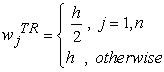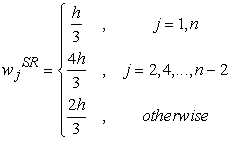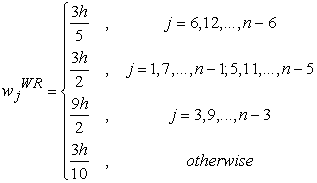The number of sub-intervals “n-1” in TR, SR and WR has to be an odd number, an even number and a multiple of six, respectively. The approximations by TR and SR in each sub-interval are respectively linear and quadratic. However, Weddle’s Rule, being a sixth order quadrature method approximates integral in (1) by a sixth order polynomial in each sub-interval, hence is assumed to provide closer approximation for solution of (1) than lower order quadrature rules.

Using (10) with respective weights defined for TR, SR and WR and evaluating it at n equally-spaced quadrature points, gives: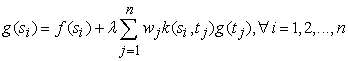(11)

Let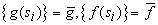and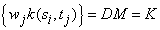, where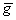and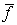are vectors of order n, D is a diagonal matrix of order n having the weights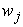’s on leading diagonal, M is an nth order square matrix of kernels and K is product of D and M. System (11), likewise (2), in associated matrix form for the approximate solutionbecomes: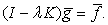(12)

The coefficient matrix “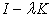” in (12) is usually invertible when the parameteris not very close to an eigen value of (1) 29. Finally (12) gives: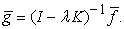(13)

Equation (13) provides open solution to (1) at n quadrature points. However, to attain solution of (1) in closed form, simple polynomial interpolation is not preferred at all, as it destroys the accuracy so obtained 29. In fact Nystrom’s Interpolation method is applied which utilizes (10) as an interpolation formula to obtain closed form solutions without losing the obtained accuracy.

Remark 1. It is important to mention that theory of construction, existence and uniqueness along with the analysis of convergence, stability and error bounds to solution of equations of type (1) by the polynomial collocation and the presented uniformly-spaced quadrature methods are well developed. For details refer 2, 5, 7, 8, 9.

### 3. Numerical Experiments and Calculations

In this work performance of discussed methods to solve (1) and necessary comparisons are generated using MATLAB (R2011). For this graphing tools and several built in functions have been modified accordingly and much help is sought from 32.

The methods discussed in preceding section have been employed on following test problems of type (1) in this section:

Example 1.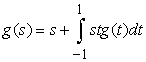.

Analytic solution of Example 1 is: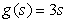. 9

Example 2.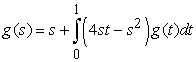and the exact solution is: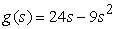. 14, 16

Example 3.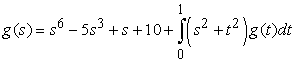. Exact solution is: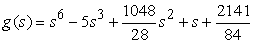. 14, 16

Example 4.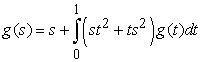.

Analytic solution is: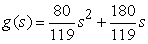. 2, 9

Example 5.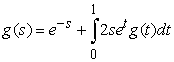. Exact solution is: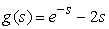. 3

Polynomial collocation method has been used with n = 3 to approximate solution of Examples 1-5 by means of polynomials of order at most 2 using (6) in the form: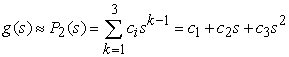(14)

through (7), assuming that the associated residual term vanishes at three collocation points s = -1, s = 0 and s = 1 (arbitrarily chosen). The corresponding coefficients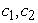and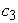in collocations approximations for these test problems 1-5 are summarized in Table 1.

Implementation of TR, SR and WR is made using corresponding weights through (11) for Examples 1-5 at seven quadrature points (i.e. with n = 7), in fact, dividing corresponding intervals of integration in into six sub-intervals of same length. It should be noted that increasing quadrature points was necessary here, as with even n =7, the exact solutions have been recovered in few examples and the over-all accuracy is found satisfactory. This is the main feature of proposed Nystrom’s procedure to extract closed form solutions. The resulting square system (12) is then solved by analytical methods as described in (13). The closed form approximations by TR, SR and WR for Problems 1-5 using Nystrom’s Interpolation formula (10) along with Polynomial Collocation approximations of order at most 2 are summarized in Table 2 along with exact solutions.

Finally, Table 3 exhibits comparison of absolute errors among the solutions by TR, SR, WR, Col methods versus the exact solutions for all test problems at seven quadrature points. Figure 1Figure 5 present error plots associated with used methods with errors highlighted at seven quadrature points for Problems 1-5, respectively.

• Figure 1. Error Plots for TR, SR, WR and Col. Methods for Test Problem # 01
• Figure 2. Error Plots for TR, SR, WR and Col. Methods for Test Problem # 02
• Figure 3. Error Plots for TR, SR, WR and Col. Methods for Test Problem # 03
• Figure 4. Error Plots for TR, SR, WR and Col. Methods for Test Problem # 04
• Figure 5. Error Plots for TR, SR, WR and Col. Methods for Test Problem # 05

### 4. Results and Discussion

Different sort of integral equations of type (1) in Examples 1-5 have been presented in detail in previous section along with their solution in closed forms using the discussed methods with error plots between exact and approximated solutions for illustrations. Examples 1-5 were intentionally selected to indulge equations of type (1) whose exact solutions are either polynomials of order one (Example 1), two (Examples 2, 4), six (Example 3) or even involving exponential function (Example 5); to better testify and compare the efficiency of discussed methods.

Examples show that the approximate solution by Polynomial collocation method coincides with the exact solution in cases where exact solution itself is a polynomial of order at most 2 (refer Examples 1, 4). But errors associated with Collocation method for Example-2 through Figure 2 do not tend to zero, which reflects that the quadratic approximation by collocation method may not provide satisfactory accuracy even when the exact solution is quadratic. The method has worked better than or equivalent to TR in some cases (see Table 3: Examples-1, 4 and 5). The solution by collocation was better than that of SR solution only for Example-4 (see Table 3: Example-4). An important result to be noticed is that for Examples 2, 3 and 5 the collocation solution is not well-conditioned as solution doesn’t converge to the exact at selected quadrature points. In view of Figure 2, Figure 3 and Figure 5 it is evident that the inefficient solutions are resulted due to ill-conditioned systems of algebraic equations caused while collocation discretization of the example problems. Thus, for the same basis set used, collocation method doesn’t provide well-conditioned discretization.

On the other hand, uniformly-spaced quadrature methods: SR and WR have shown better results as compared to TR for all test problems (see Figure 1Figure 5 and Table 3). The closed form solutions by SR for Examples 1-2 coincide with, and approximate well the exact solutions for rest of the problems (refer Table 2 and Figure 1Figure 5). It is very important to note that the WR has resulted in zero discrepancy in all examples because closed form solutions by WR (see Table 2) are simply the exact solutions, except in Example-3. The exact solution of Example-3 is a sixth order polynomial and WR has yielded an approximate solution with smaller errors compared to other methods (Table 3). In particular, SR for Example-3 has resulted errors of order 10-2 which with WR are of order 10-4 at most of the quadrature points, suggesting better approximation than SR. So, WR results in exact closed form solutions using Nystrom’s Interpolation in most of the cases and gives better results than other discussed methods when the exact solution is a higher degree polynomial.

Consequently except the TR, which is a linear approximation, higher order quadrature methods SR and WR provide well-conditioned systems through discretization and hence are better than the collocation method under the similar conditions to solve Integral equations of type (1).

### 5. Conclusion

Numerical solution of non-homogeneous linear Fredholm integral equation of second kind for the case of non-singular kernel is presented by polynomial collocation and uniformly spaced quadrature rules. Results obtained by implementation of discussed method on various sort of test problems have been compared. Nystrom’s Interpolation method has also been used to provide closed form solutions by quadrature methods. It is observed from through obtained results that the polynomial collocation method with n = 3 has shown good results for some problems whose exact solution is a polynomial of at most second order. However, in some problems, collocation method yields ill-conditioned systems through discretization even with the same basis set. Under similar conditions, quadrature methods: SR and WR have shown better results as compared to TR and Col. in all cases. The WR approximation versus the exact solution resulted in zero discrepancy for almost all test problems and otherwise has shown smaller errors compared to SR. It can be concluded that the WR results in exact closed form solutions using Nystrom’s Interpolation and gives better results than other methods when the exact solution is a higher degree polynomial.

### Acknowledgements

This work is dedicated to Prof. Manzoor Ahmed Memon (Late), Associate Professor, IMCS, University of Sindh, Jamshoro, Pakistan who initially motivated the author for research on these methods. Author is also thankful to Dr. Ayaz Keerio, PhD, University of Sussex, UK, for going through the work to make its current version more readable. Finally, author is thankful to Prof. Dr. Ingr. Karem Boubker from Tunisia for reviewing this work before, and a few months later he left this world.

### ReferencesThis work is licensed under a Creative Commons Attribution 4.0 International License. To view a copy of this license, visit http://creativecommons.org/licenses/by/4.0/